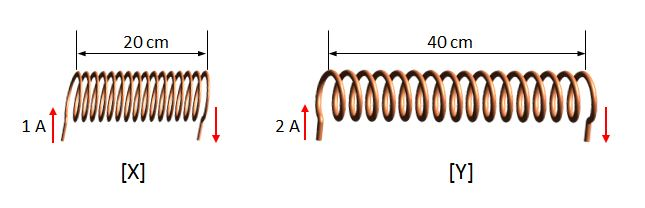# Two solenoidsThere are two solenoids X and Y with lengths $20\text{ cm}$ and $40\text{ cm},$ respectively. If the current intensities flowing in X and Y are $1\text{ A}$ and $2\text{ A},$ respectively, what is the ratio of the intensities of the magnetic fields induced inside X and Y?

The number of turns of the two solenoids are the same.

×